# Program to check Nelson Number in Java

In this tutorial you will learn about the Program to check Nelson Number in Java and its application with practical example.

In this tutorial, we will learn to create a Java Program to check Nelson Numbers in Java using Java programming.

## Prerequisites

Before starting with this tutorial we assume that you are best aware of the following Java programming topics:

• Java Operators.
• Basic Input and Output function in Java.
• Class and Object in Java.
• Basic Java programming.
• If-else statements in Java.
• For loop in Java.

## What is Nelson Number

The number “111” is called “Nelson Number” after the name of sir “Admiral Nelson“,because he had only had “One Eye, One Arm, One Leg” at the end of his life.Double  of Nelson number called  Double Nelson (222), triple Nelson (333), and so on..

## Program to check Nelson Number in Java

In this our program we will find given number is a Nelson number or not in using java programming. We would first declared and initialized the required variables. Next, we would prompt user to input the a number.Later we  find number is Nelson number or not let’s have a look at the code.

## Output

Nelson number.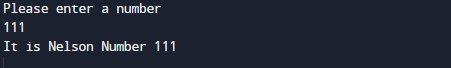Not a nelson number.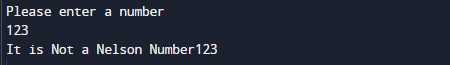In the above program, we have first declared and initialized a set variables required in the program.

• number= it will hold entered number.

After declaring variables in the next statement user will be prompted to enter a value and  which will be assigned to variable ‘number’.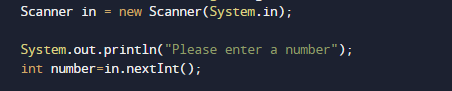And after that we check condition for Nelson number if condition get satisfied it means the given number is Nelson number if not then it is not  Nelson number.

Condition for Nelson number.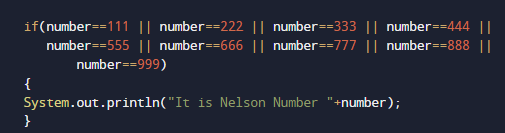If the above Condition satisfied the given number by user is a Nelson number.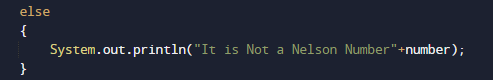If not then the given number is not a Nelson number.

In this tutorial we have learn about the Program to check Nelson Number in Java and its application with practical example. I hope you will like this tutorial.